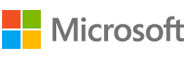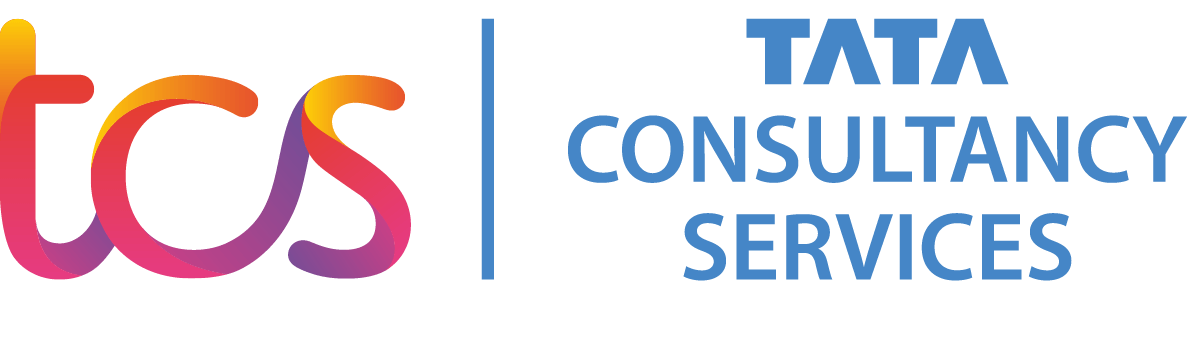New update is available. Click here to update.
Topics

# Reverse DLL nodes in groups

Moderate0/80
Average time to solve is 10m+2 more companies

## Problem statement

You are given a Doubly Linked List of integers and a positive integer 'K' representing the group size. Modify the linked list by reversing every group of 'K' nodes in the linked list.

``````A doubly linked list is a type of linked list that is bidirectional, that is, it can be traversed in both directions, forward and backward.
``````
###### Note:
``````If the number of nodes in the list or in the last group is less than K, just reverse the remaining nodes.
``````
Example:
``````Linked list: 8 9 10 11 12
K: 3

Output: 10 9 8 12 11

We reverse the first K (3) nodes. Now, since the number of nodes remaining in the list (2) is less than K, we just reverse the remaining nodes (11 and 12).
``````
Detailed explanation ( Input/output format, Notes, Images )
Constraints:
``````1 <= T <= 10
1 <= N <= 5 * 10^4
1 <= K <=  10^5
-10^3 <= data <= 10^3 and data != -1

Time Limit: 1 sec
``````
Sample Input 1:
``````1
1 2 3 4 5 6 7 -1
2
``````
Sample Output 1:
``````2 1 4 3 6 5 7 -1
``````
Explanation of sample input 1:
``````In the given linked list, we have to reverse the first two nodes, then reverse the next two nodes, and so on… until all the nodes are processed in the same way.
The modified linked list after the above process is 2 1 4 3 6 5 7 -1
``````
Sample Input 2:
``````2
5 10 -6 4 7 -1
3
10 20 30 40 50 -1
1
``````
Sample Output 2:
``````-6 10 5 7 4 -1
10 20 30 40 50 -1
``````Console